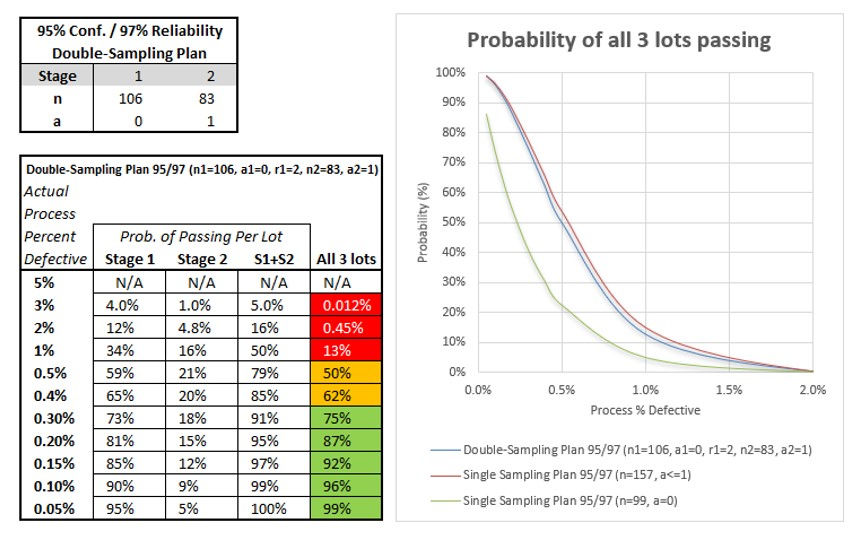top of page

# The REAL odds of passing a Process Validation - Part 4

In Part 3 of this series we concluded by saying that, unless the lot sizes used in production are very large, we seldom use plans greater than a<=1 in a process validation (PQ) study. The sample size, cost and duration of the study become too great compared to the benefits - and there are better alternatives.

Double-Sampling Plans are one such alternative. They increase the probability of passing the study while keeping the effective sample size comparable to a=0 plans.

As an example, take an attribute Double-Sampling plan that may be used to achieve 95% confidence / 97% reliability with the following parameters:

n1 = 106, a1= 0, r1 = 2

n2 = 83, a2 = 1

The plan works like this:

• Take a first sample (stage 1) of 106 units (n1=106); if there are 0 defective units in the sample, the acceptance criterion (a1=0) has been satisfied (pass) and inspection stops. If there are 2 defective units found in the sample, the rejection criterion (r1=2) has been satisfied (fail) and inspection stops. If there is only 1 defective unit found (between a1 and r1), proceed to the second stage.

• Second stage (stage 2), take an additional sample of 83 units; if there is only 1 defective unit (a2=1) found in both stages (106+83 units), the acceptance criterion has been satisfied. Otherwise, the acceptance criterion has NOT been satisfied (fail).

If we assume, as we have for Parts 1 - 3 of this post, that the sample sizes and acceptance criteria above are applied to each lot, the probability of all 3 lots passing vs. actual process percent defective is shown below.Notice how similar the probabilities for the Double-Sampling plan and for the a<=1 plan are. Also, when the process percent defective is <= 0.4%, the ratio of the probabilities of passing on the first stage compared to the second stage is >= 4X.

This means most of the time using a Double-Sampling Plan will result in each lot passing during the first stage as compared to the second stage, and we won't need to take additional samples.

Using double-sampling, the study will be completed faster and with considerably less inspection than with an a<=1 plan.

Note: we need to specify ahead of time (i.e., in the protocol) that a double-sampling plan will be used and its parameters. Do NOT specify a single sampling plan and then inspect additional parts during study execution!

## Conclusion

We have seen in this post series that when considering studies where the confidence/reliability, sample size and acceptance criterion are applied to each lot, the process percent defective must be much lower (<1/20th) than what is indicated by the reliability when using accept on zero failures (a=0) sampling plans.

Accept on at most 1 failure (a<=1) plans provide a much higher probability of passing the study, but result in a significant increase in the sample size needed (+58%).

Use of double-sampling plans provide the higher probability of passing that a<=1 plans do, while having effective sample sizes closer to a=0 sampling plans. For this reason, it is highly recommended to use double-sampling plans whenever possible for attribute analysis studies.

<If you liked this post, click the small heart icon below (it lets me know to create more content like this.)>

124 views

## Related Posts

See All

BLOG

New posts added regularly. To be among the first to know, subscribe.

bottom of page